Processing ......FreeComputerBooks.com Free Computer, Mathematics, Technical Books and Lecture Notes, etc.

Machine Learning
Related Book Categories:
 Artificial Intelligence (AI) R Programming Computer and Machine Vision Data ScienceProbability and Stochastic Processes Optimization and Approximation Mathematical Logic
•Deep Learning and Neural Networks

A collection of books related to Deep Learning and Neural Networks, including (but not limited to): Artificial Neural Networks (ANN), Recurrent Neural Networks (RNN), Convolutional Neural Network (CNN, or ConvNet), GANs, etc.

•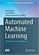Automated Machine Learning: Methods, Systems, Challenges

This book presents the first comprehensive overview of general methods in Automated Machine Learning (AutoML), collects descriptions of existing systems based on these methods, and discusses the first series of international challenges of AutoML systems.

•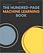The Hundred-Page Machine Learning Book (Andriy Burkov)

Everything you really need to know in Machine Learning in a hundred pages! This book provides a great practical guide to get started and execute on ML within a few days without necessarily knowing much about ML apriori.

•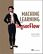Machine Learning with TensorFlow (Nishant Shukla)

This book gives readers a solid foundation in machine-learning concepts plus hands-on experience coding TensorFlow with Python. You'll learn the basics by working with classic prediction, classification, and clustering algorithms.

•Foundations of Machine Learning (Mehryar Mohri, et al)

This book is a general introduction to machine learning. It covers fundamental modern topics in machine learning while providing the theoretical basis and conceptual tools needed for the discussion and justification of algorithms.

•A Course in Machine Learning (Hal Daume III)

This is a set of introductory materials that covers most major aspects of modern machine learning (supervised learning, unsupervised learning, large margin methods, probabilistic modeling, learning theory, etc.).

•Understanding Machine Learning: From Theory to Algorithms

This book explains the principles behind the automated learning approach and the considerations underlying its usage. It provides an extensive theoretical account of the fundamental ideas underlying machine learning and the mathematical derivations that transform these principles into practical algorithms.

•An Introduction to Statistical Learning (Gareth James, et al)

It provides an accessible overview of the field of statistical learning, an essential toolset for making sense of the vast and complex data sets that have emerged in fields ranging from biology to finance to marketing to astrophysics in the past twenty years.

•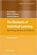The Elements of Statistical Learning: Data Mining, Inference, etc.

This book describes the important ideas in these areas in a common conceptual framework. While the approach is statistical, the emphasis is on concepts rather than mathematics. Many examples are given, with a liberal use of color graphics.

•Statistical Learning and Sequential Prediction

This book focuses on theoretical aspects of Statistical Learning and Sequential Prediction, a unified approach to analyzing learning in both scenarios, brings together ideas from probability and statistics, game theory, algorithms, and optimization.

•Interpretable Machine Learning: Black Box Models Explainable

This book explains to you how to make (supervised) machine learning models interpretable. The book focuses on machine learning models for tabular data (also called relational or structured data) and less on computer vision and NLP tasks.

•Machine Learning for Cyber Physical Systems (Jurgen Beyerer)

This book presents new approaches to Machine Learning for Cyber Physical Systems, experiences and visions. Typical Cyber Physical Systems applications are condition monitoring, predictive maintenance, image processing and diagnosis.

•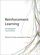Reinforcement Learning: An Introduction, Second Edition

It provides a clear and simple account of the key ideas and algorithms of reinforcement learning that is accessible to readers in all the related disciplines. Focuses on core online learning algorithms, with the more mathematical material set off in shaded boxes.

•Algorithms for Reinforcement Learning (Csaba Szepesvari)

This book focuses on those algorithms of reinforcement learning that build on the powerful theory of dynamic programming. It gives a fairly comprehensive catalog of learning problems, describe the core ideas, note a large number of state of the art algorithms, followed by the discussion of their theoretical properties and limitations.

•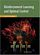Reinforcement Learning and Optimal Control (Dimitri Bertsekas)

The purpose of the book is to consider large and challenging multistage decision problems, which can be solved in principle by dynamic programming and optimal control, but their exact solution is computationally intractable.

•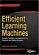Efficient Learning Machines: Theories, Concepts, and Applications

It weaves together the theoretical exposition, design principles, and practical applications of efficient machine learning, aims to equip engineers, students of engineering, and system designers to design and create new and more efficient machine learning systems.

•Bayesian Reasoning and Machine Learning (David Barber)

This practical introduction is ideally suited to computer scientists without a background in calculus and linear algebra. You'll develop analytical and problem-solving skills that equip them for the real world. Numerous examples and exercises are provided.

•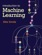Introduction to Machine Learning (Alex Smola, et al)

This book is a introductory textbook on the subject, discussesing many methods from different fields, including statistics, pattern recognition, neural networks, artificial intelligence, signal processing, control, and data mining.

•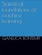Statistical Foundations of Machine Learning (Gianluca Bontempi)

This book aims to present the statistical foundations of machine learning intended as the discipline which deals with the automatic design of models from data. All the examples are implemented in the statistical programming language R.

•Boosting: Foundations and Algorithms (Robert E. Schapire, et al)

This book, written by the inventors of the Boosting method, brings together, organizes, simplifies, and substantially extends two decades of research on boosting, presenting both theory and applications in a way that is accessible to readers from diverse backgrounds.

•Azure Cognitive Services Succinctly (Ed Freitas)

A practical guide to working with cognitive APIs developed by Microsoft and provided with the Azure platform to developers and businesses. It delivers an accessible guide to integrating computer vision, decision-making, speech, and more into your apps.

•Support Vector Machines Succinctly (Alexandre Kowalczyk)

This book guides readers through the building blocks of Support Vector Machines (SVMs), from basic concepts to crucial problem-solving algorithms. It also includes numerous code examples and a lengthy bibliography for further study.

•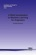A Brief Introduction to Machine Learning for Engineers

This book aims at providing an introduction to key concepts, algorithms, and theoretical frameworks in machine learning, including supervised and unsupervised learning, statistical learning theory, probabilistic graphical models and approximate inference.

•The LION Way: Machine Learning Plus Intelligent Optimization

This book captures the state of the art of the interaction between optimization and machine learning in a way that is accessible to researchers in both fields. Optimization approaches have enjoyed prominence in machine learning because of their wide applicability and attractive theoretical properties.

•Introduction to Machine Learning (Amnon Shashua)

This book will be exploring machine learning, the concepts that run these technologies and by the time you get to the end you will have more knowledge than many and will be equipped to start building your own applications.

•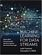Machine Learning for Data Streams: Practical Examples in MOA

This book presents algorithms and techniques used in data stream mining and real-time analytics. Taking a hands-on approach, it demonstrates the techniques using MOA (Massive Online Analysis), a popular, freely available open-source software framework, allowing readers to try out the techniques after reading the explanations.

•Gaussian Processes for Machine Learning (Carl E. Rasmussen)

This book provides a long-needed systematic and unified treatment of theoretical and practical aspects of Gaussian Processes (GPs) in machine learning. It deals with the supervised-learning problem for both regression and classification.

•Machine Learning: The Complete Guide (Wikipedia)

This book provides an extensive theoretical account of the fundamental ideas underlying machine learning and the mathematical derivations that transform these principles into practical algorithms. It also covers a wide array of central topics that have not been addressed by other books.

•Machine Learning and Data Mining (Aaron Hertzmann)

This is an introduction to the main issues associated with the basics of machine learning and the algorithms used in data mining. It offers a grounding in machine learning concepts as well as practical advice on techniques in real-world data mining.

•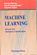Machine Learning, Neural and Statistical Classification (D. Michie)

Statistical, machine learning and neural network approaches to classification are all covered in this book to provide an objective assessment of the potential for machine learning algorithms in solving significant commercial and industrial problems, widening the foundation for exploitation of these and related algorithms.

•Deep Learning (Ian Goodfellow, et al)

Written by three experts, this is the only comprehensive book on the subject. It offers mathematical and conceptual background, covering relevant concepts in linear algebra, probability theory and information theory, numerical computation, and machine learning.

•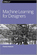O'Reilly® Machine Learning for Designers (Patrick Hebron)

This book not only introduces you to contemporary machine learning systems, but also provides a conceptual framework to help you integrate machine-learning capabilities into your user-facing designs, using tangible, real-world examples.

•O'Reilly® The Future of Machine Intelligence (David Beyer)

This exclusive report unpacks concepts and innovations that represent the frontiers of ever-smarter machines. You’ll get a rare glimpse into this exciting field through the eyes of some of its leading minds.

•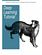Deep Learning Tutorials (LISA Lab)

The tutorials presented here will introduce you to some of the most important deep learning algorithms and will also show you how to run them using Theano. Theano is a python library that makes writing deep learning models easy, and gives the option of training them on a GPU.

•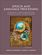Speech and Language Processing (Dan Jurafsky, et al)

This text takes an empirical approach to the subject, based on applying statistical and other machine-learning algorithms to large corporations. It describes a unified vision of speech and language processing. Emphasis is on practical and scientific applications.

•New Advances in Machine Learning (Yagang Zhang)

This book provides an up-to-date and systematical introduction to the principles and algorithms of machine learning, as well as a good introduction to many approaches of machine learning, and the source of useful bibliographical information.

•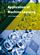Application of Machine Learning (Yagang Zhang)

Present the latest applications of machine learning, which mainly include: speech recognition, traffic and fault classification, surface quality prediction in laser machining, network security and bioinformatics, enterprise credit risk evaluation, and so on.

•Numerical Algorithms: Computer Vision, Machine Learning, etc.

This book presents a new approach to numerical analysis for modern computer scientists, covers a wide range of topics - from numerical linear algebra to optimization and differential equations - focusing on real-world motivation and unifying themes.

•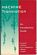Machine Translation: An Introductory Guide (Doug Arnold, et al)

This introductory book looks at all aspects of Machine Translation: covering questions of what it is like to use a modern Machine Translation system, through questions about how it is done, to questions of evaluating systems, and what developments can be foreseen.

•Inductive Logic Programming: Techniques and Applications

This book is an introduction to inductive logic programming (ILP), which aims at a formal framework as well as practical algorithms for inductively learning relational descriptions in the form of logic programs.

•Learning Deep Architectures for AI (Yoshua Bengio)

This book discusses the motivations for and principles of learning algorithms for deep architectures. By analyzing and comparing recent results with different learning algorithms for deep architectures, explanations for their success are proposed.

•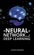Neural Networks and Deep Learning (Michael Nielsen)

Neural networks and deep learning currently provide the best solutions to many problems in image recognition, speech recognition, and natural language processing. This book will teach you the core concepts behind neural networks and deep learning.

•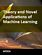Theory and Novel Applications of Machine Learning (Meng Joo Er)

This books reports the latest developments and futuristic trends in Machine Learning. It involves broad topics including Fuzzy Logic, Neural Networks (NNs), Evolutionary Algorithms (EAs), Probability and Statistics, Decision Trees, etc.

•Machine Learning Using C# Succinctly (James McCaffrey)

This book shows several different approaches to applying machine learning to data analysis and prediction problems. It also demonstrates different clustering and classification techniques, and explains how effective these techniques can be.

•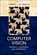Computer Vision: Models, Learning, and Inference (Simon Prince)

The book starts from the basics of probability and model fitting and works up to real examples that the reader can implement and modify to build useful vision systems. The detailed methodological presentation is useful for practitioners of computer vision.

•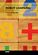Robot Learning (Suraiya Jabin)

It gives a focused introduction to the primary themes in a Robot learning course and demonstrates the relevance and practicality of various Machine Learning algorithms to a wide variety of real-world applications from evolutionary techniques to reinforcement learning, classification, control, uncertainty and many other important fields.

•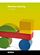Machine Learning (Yagang Zhang)

This book presents today's state and development tendencies of machine learning. Taking into account the large amount of knowledge about machine learning and practice presented in the book, it is divided into three major parts: Introduction, Machine Learning Theory and Applications.

•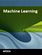Machine Learning (Abdelhamid Mellouk, et al)

The book provides an extensive theoretical account of the fundamental ideas underlying machine learning and the mathematical derivations that transform these principles into practical algorithms.

•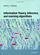Information Theory, Inference, and Learning Algorithms (D MacKay)

This textbook introduces Information theory in tandem with applications. Information theory is taught alongside practical communication systems, such as arithmetic coding for data compression and sparse-graph codes for error-correction.

•AI Algorithms, Data Structures, and Idioms in Prolog, Lisp, and Java

Illustrateing how to program AI algorithms in Lisp, Prolog, and Java. Topics include: simple production-like system based on logic, logic-based learning, and natural language parsing.

•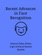Recent Advances in Face Recognition (Kresimir Delac, et al)

This book provides a broad overview on face recognition and identified trends for future developments and the means for implementing robust systems..

•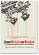From Bricks to Brains: Embodied Cognitive Science of LEGO Robots

This book introduces embodied cognitive science and illustrates its foundational ideas through the construction and observation of LEGO Mindstorms robots.

•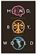Mind, Body, World: Foundations of Cognitive Science (M. Dawson)

Intended to introduce the foundations of cognitive science, this book addresses a number of questions currently being asked by those practicing in the field of cognitive science. It highlights the fundamental tensions and lines of fragmentation of cognitive science.

•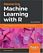Mastering Machine Learning with R (Cory Lesmeister)

This book will teach you advanced techniques in machine learning with the latest code in R. You will delve into statistical learning theory and supervised learning; design efficient algorithms; learn about creating Recommendation Engines; and more.

•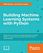Building Machine Learning Systems with Python (Willi Richert)

Featuring a wealth of real-world examples, this book provides gives you with an accessible route into Python machine learning. You'll learn everything you need to tackle the modern data deluge - by harnessing the unique capabilities of Python.

•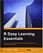R Deep Learning Essentials (Joshua F. Wiley)

This book offers you the essentials every developer needs to get started - from the packages you need to have for your side, building models related to neural networks, prediction, and deep prediction, to fine tuning and optimizing everything you have.

•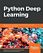Python Deep Learning (Valentino Zocca, et al)

Take your machine learning skills to the next level by mastering Deep Learning concepts and algorithms using Python. This book will give you all the practical information available on the subject, including the best practices, using real-world use cases.

Book CategoriesAll CategoriesRecent BooksIT Research LibraryMiscellaneous Books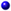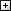Computer LanguagesComputer ScienceData Science/DatabasesElectronic EngineeringJava and Java EE (J2EE)Linux and UnixMathematicsMicrosoft and .NETMobile ComputingNetworking and CommunicationsSoftware EngineeringSpecial TopicsWeb Programming
Other Categories